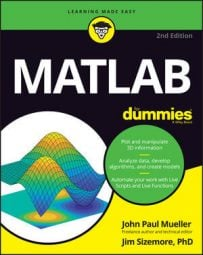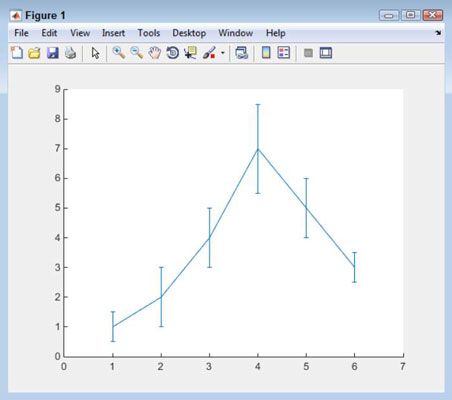##### MATLAB For DummiesMATLAB can help you find data deviations. An error bar chart shows the level of confidence in each data point along a line. What you see is the transition between data points as a solid line, and then a bar that shows the amount of deviation, both positive and negative, from that point.

A viewer can tell whether the data is truly accurate or simply a guess by looking at the amount of deviation for particular points along the line. This kind of plot helps with analysis in determining whether the data is solid enough to rely on or whether additional input is needed to obtain a better estimate. The following steps help you create an error bar plot:

1. Type YSource = [1, 2, 4, 7, 5, 3]; and press Enter.

These values represent the actual data points. They tell the viewer what you think the actual values might be.

2. Type ESource = [.5, 1, 1, 1.5, 1, .5]; and press Enter.

These values represent the amount of error for each data point. This is the plus or minus amount and tells the viewer how confident you are about the values in YSource.

3. Type EBar1 = errorbar(YSource, ESource); and press Enter.

This step creates the error bar plot. Notice that each data point has an error bar associated with it, showing the potential amount of deviation for that data point.